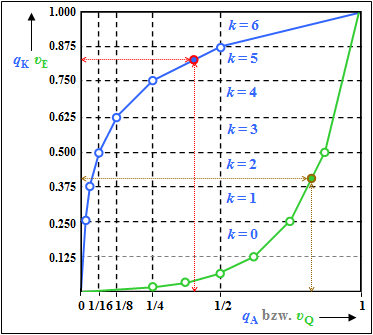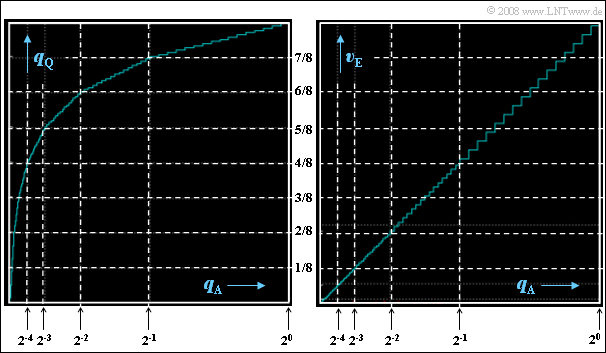# Exercise 4.5: Non-Linear Quantization

To investigate  "non-linear quantization"  we start from the outlined system model.

• We disregard the influence of the channel and the PCM coding or decoding.
• Thus,  $v_{\rm Q}(ν \cdot T_{\rm A}) = q_{\rm Q}(ν \cdot T_{\rm A})$  always applies,  whereby the time specification  $ν \cdot T_{\rm A}$  is omitted in the following.

By comparing one output variable with one input variable at the same time,  it is possible to determine the influence

• of the compressor   ⇒    $q_{\rm K}(q_{\rm A})$,
• of the linear quantizer   ⇒    $q_{\rm Q}(q_{\rm K})$,
• of the non-linear quantizer   ⇒    $q_{\rm Q}(q_{\rm A})$,
• of the expander   ⇒    $v_{\rm E}(v_{\rm Q})$,  and
• of the overall system   ⇒    $v_{\rm E}(q_{\rm A})$.

The following assumptions are made:

• All samples  $q_{\rm A}$  are in the value range  $±1$  .
• The  (linear)  quantizer works with  $M = 256$  quantization levels,  which are marked with  $μ = 0$  to  $μ = 255$ .
• For compression,  the so-called  "13-segment"  characteristic is used.

This means:

• In the range  $|q_{\rm A}| ≤ 1/64$  holds  $q_{\rm K} = q_{\rm A}$.
• For  $q_{\rm A} > 1/64$,  there are the following six additional ranges  $(k = 1$, ... , $6)$  of the compressor characteristic:
⇒   range $k\hspace{0.3cm}{\rm (if}\hspace{0.3cm} 2^{k-7}< q_{\rm A} \le 2^{k-6}) \hspace{0.05cm}$   ⇒   $q_{\rm K}(q_{\rm A}) = 2^{4-k} \cdot q_{\rm A} + {k}/{8}.$
• Another six domains exist for negative  $q_{\rm A}$  values with  $k = -1$, ... , $-6$,  which are point-symmetric with respect to the origin.
However,  these are not considered further in this exercise.

Hints:

### Questions

1

If  $q_{\rm A} = 0.4$:   What is the output value  $q_{\rm K}$  of the compressor?

 $q_{\rm K} \ = \$

2

To which quantization interval  $μ$  does  $q_{\rm A} = 0.4$  belong?

 $\mu \ = \$

3

Which quantization value  $q_{\rm Q}$  belongs to  $q_{\rm A} = 0.4$?

 $q_{\rm Q} \ = \$

4

In contrast,  what quantization value  $q_{\rm Q}$  belongs to  $q_{\rm A} = 0.04$?

 $q_{\rm Q} \ = \$

5

At the receiver,  the input value is  $v_{\rm Q} = 211/256 ≈ 0.824$.  What value  $v_{\rm E}$  does the expander provide?

 $v_{\rm E} \ = \$

6

What are the properties of the  "non-linear quantizer characteristic"  $q_{\rm Q}(q_{\rm A})$ ?

 The characteristic  $q_{\rm Q}(q_{\rm A})$  approximates the compressor characteristic in steps. The characteristic  $q_{\rm Q}(q_{\rm A})$  approximates the angle bisector in steps. The step width is the same in all segments  $($except for  $k = 0)$ . The step height is equal in all segments  $($except for  $k = 0)$ .

7

What are the properties of the  "overall system characteristic"  $v_{\rm E}(q_{\rm A})$ ?

 The characteristic  $v_{\rm E}(q_{\rm A})$  approximates the compressor characteristic in steps. The characteristic  $v_{\rm E}(q_{\rm A})$  approximates the angle bisector in steps. The step width is the same in all segments  $($except for  $k = 0)$ . The step height is equal in all segments  $($except for  $k = 0)$ .

### Solution

#### Solution

(1)  The sample  $q_{\rm A} = 0.4$  belongs to the segment  $k = 5$  covering the range  $1/4 < q_{\rm A} ≤ 1/2$.  From the given equation it follows that with  $k = 5$:

$$q_{\rm K}(q_{\rm A}) = 2^{4-k} \cdot q_{\rm A} + {k}/{8}={1}/{2}\cdot 0.4 + {5}/{8} \hspace{0.15cm}\underline {= 0.825}\hspace{0.05cm}.$$

(2)  The input value of the linear quantizer is now  $q_{\rm K} = 0.825$,  so the following calculation applies:

$${105}/{128} < q_{\rm K} = 0.825 \le {106}/{128}\hspace{0.3cm}\Rightarrow \hspace{0.3cm} m = 105 \hspace{0.3cm}\Rightarrow \hspace{0.3cm} \mu = 128 + 105\hspace{0.15cm}\underline { = 233} \hspace{0.05cm}.$$

(3)  According to the specification page, the quantization interval  $μ = 128 + m$  is given by the value  $q_{\rm Q} = 1/256 + m/128$.  With  $m = 105$  it follows:

$$q_{\rm Q} = \frac{1}{256} + \frac{105}{128} \hspace{0.15cm}\underline {\approx 0.824} \hspace{0.05cm}.$$

(4)  According to the sample solution to subtask  (3)  with the input value  $q_{\rm A} = 0.04$:

$$\frac{1}{32} < q_{\rm A} \le \frac{1}{16}\hspace{0.3cm}\Rightarrow \hspace{0.3cm} k = 2 \hspace{0.3cm}\Rightarrow \hspace{0.3cm} q_{\rm K} = 2^2 \cdot 0.04 + \frac{2}{8}= 0.41$$
$$\Rightarrow \hspace{0.3cm}\frac{52}{128} < q_{\rm K} = 0.41 \le \frac{53}{128}\hspace{0.3cm}\Rightarrow \hspace{0.3cm} m = 52 \hspace{0.3cm}\Rightarrow \hspace{0.3cm} \mu = 128 + 52 = 180\hspace{0.3cm} \Rightarrow \hspace{0.3cm}q_{\rm Q} = \frac{1}{256} + \frac{52}{128} \hspace{0.15cm}\underline {= 0.41} \hspace{0.05cm}.$$Characteristics of compressor (blue) & expander (green)

(5)  We are looking for the solution in several steps:

• In the compressor:   $q_{\rm A} = 0.4$  led to the initial value  $q_{\rm K} = 0.825$  and after quantization to the value  $q_{\rm Q} = 0. 824$   ⇒   see subtasks  (1)  and  (3).  ⇒   red marks in the graph.
• On the receiver side,  this results in  $v_{\rm Q} = 0.824$  approximately back to  $v_{\rm E} ≈ 0.4$   ⇒   brown marks in the graph.
• However,  due to quantization,  this is only an approximation.  Exactly:
$$v_{\rm E} = 0.25 + \frac{0.824-0.750}{0.875-0.750} \cdot 0.25 \hspace{0.15cm}\underline {= 0.398} \hspace{0.05cm}.$$

This calculation process can be understood from the graph.

Although the expander characteristic  $v_E(υ_{\rm Q})$  is equal to the inverse function of the compressor characteristic  $q_K(q_{\rm A})$  an error results because the input  $v_{\rm Q}$  of the expander is discrete in value  (influence of quantization).

(6)  Correct are the  statements 1 and 4,  as can be verified by the following left graph:13-segment characteristic curves: left:  $q_{\rm Q}(q_{\rm A})$,           right:  $v_{\rm E}(q_{\rm A})$
• The width of each step is different in each segment.  In the outermost segment  $(k = 6)$  the step width is  $0.5/16 = 1/32$,  in the next segment  $(k = 5)$  only more  $0.25/16 = 1/64$.
• The step widths in the further segments are  $1/128 \ (k = 4)$,  $1/256 \ (k = 3)$,  $1/512\ (k = 2)$  and  $1/1024 \ (k = 1)$.
• The innermost range from  $-1/64$  to  $+1/64$  is divided into  $64$  steps,  resulting in the step width  $1/2048$.
• The step height,  on the other hand,  is constantly equal  $1/8$  divided by  $16 = 1/128$  in the segments  $k ≠ 0$  and equal  $1/256$ in the middle segment.

(7)  Correct here is  only the second statement:

• By the expander,  the quantization is now along the bisector of the angle.
• In each segment,  step width and step height are constant.
• As the right graphic shows,  however,  in the next inner segment the width and the height are only half as large.